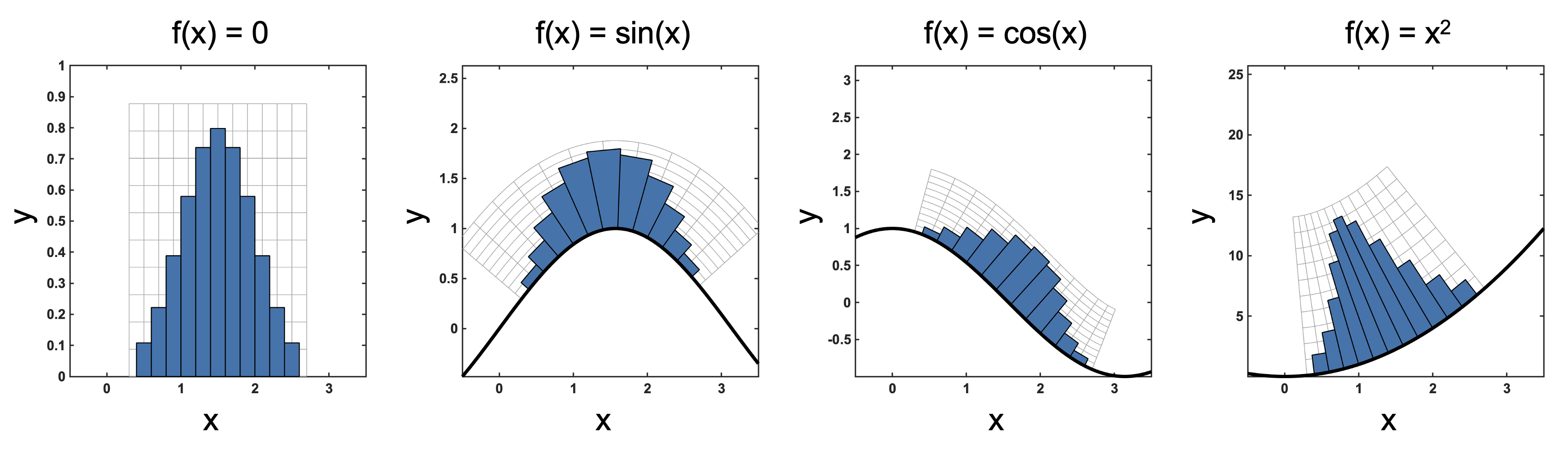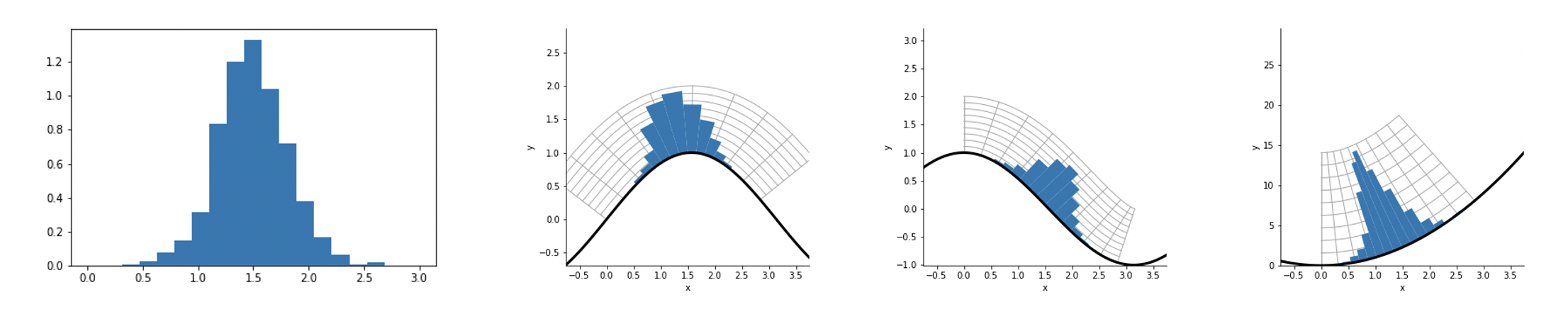## Curvogram

Version 1.0.0 (939 KB) by
Method for plotting histograms on a curve using a West Transform

Updated 26 Apr 2022

From GitHub

# Curvogram

Envisioned by Ryan Schenck with development of method from Jeffrey West.

Method for plotting histograms on a curve using a West Transform. Originally, the term 'histo' means mast, as in a sailboat mast that holds the luff of a sail. Here we call these a curvogram, which is a histogram on a curve that is given by a user defined function. The transformed coordinates are a conformal mapping to the curve/function defined by the user, termed the West Coordinate Transform.

Together, the West Coordinate Transform and the Curvogram represent a fun way to visualize high density information that is often plotted separately for sake of accuracy. However, this separation, may not be necessary if the take away for a plot is easily understood using a curvogram or the curvogram is supplemented with the original histogram elsewhere.

## Examples

Here are two versions with matlab and python code.

### MatlabHere we show the functions for a normal distribution whose mean is 1.5 and variance of 0.5. The curve functions are f(x)=sin(x), f(x)=cos(x), and f(x)=x^2.### Python

Here we show the same curve functions: f(x)=sin(x), f(x)=cos(x), and f(x)=x^2.### Important considerations:

1. The curve cannot be that complex. So simple x/y relationships work best.
2. The distributions that you plot on the curve needs to be clear, but how clear depends entirely on the steepness of the curve.
3. The plotting area must be square.

### Cite As

Alexander Anderson (2023). Curvogram (https://github.com/MathOnco/Curvogram/releases/tag/1.0.0), GitHub. Retrieved .

##### MATLAB Release Compatibility
Created with R2021b
Compatible with any release
##### Platform Compatibility
Windows macOS Linux

### Community Treasure Hunt

Find the treasures in MATLAB Central and discover how the community can help you!

Start Hunting!

#### MATLAB

Version Published Release Notes
1.0.0

To view or report issues in this GitHub add-on, visit the GitHub Repository.
To view or report issues in this GitHub add-on, visit the GitHub Repository.